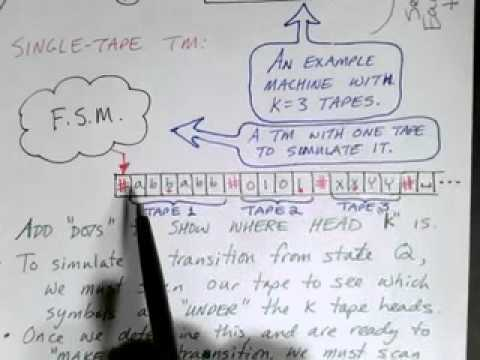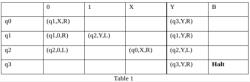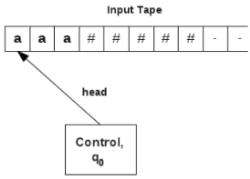Courses

# Test: Multitape Turing Machines

## 10 Questions MCQ Test Theory of Computation | Test: Multitape Turing Machines

Description
This mock test of Test: Multitape Turing Machines for Computer Science Engineering (CSE) helps you for every Computer Science Engineering (CSE) entrance exam. This contains 10 Multiple Choice Questions for Computer Science Engineering (CSE) Test: Multitape Turing Machines (mcq) to study with solutions a complete question bank. The solved questions answers in this Test: Multitape Turing Machines quiz give you a good mix of easy questions and tough questions. Computer Science Engineering (CSE) students definitely take this Test: Multitape Turing Machines exercise for a better result in the exam. You can find other Test: Multitape Turing Machines extra questions, long questions & short questions for Computer Science Engineering (CSE) on EduRev as well by searching above.
QUESTION: 1

### A turing machine with several tapes in known as:

Solution:

A multitape turing machine is an ordinary turing machine with multiple tapes. Each tape has its own head to control the read and write.

QUESTION: 2

### A multitape turing machine is ________ powerful than a single tape turing machine.

Solution:

The multitape turing machine model seems much powerful than the single tape model, but any multi tape machine, no matter how many tapes, can be simulated by single taped TM.

QUESTION: 3

### In what ratio, more computation time is needed to simulate multitape turing machines using single tape turing machines?

Solution:

Thus, multitape turing machines cannot calculate any more functions than single tape machines.

QUESTION: 4

Which of the following is true for two stack turing machines?
a) o
b)
c)
d

Solution:

Two-stack Turing machines have a read-only input and two storage tapes. If a head moves left on either tape a blank is printed on that tape, but one symbol from a “library” can be printed.

QUESTION: 5

Which of the following is not a Non deterministic turing machine?

Solution:

A read only turing machine or 2 way deterministic finite automaton is a class of model of computability that behaves like a turing machine, and can move in both directions across input, except cannot write to its input tape.

QUESTION: 6

Which of the turing machines have existential and universal states?

Solution:

ATM is divide into two sets: an existential state is accepting if some transitions leads to an accepting state; an universal state is accepting if every transition leads to an accepting state.

QUESTION: 7

Which of the following is false for Quantum Turing machine?

Solution:

‘Is a universal quantum computer sufficient’ is one of the unsolved problem from physics.

QUESTION: 8

A deterministic turing machine is:

Solution:

A deterministic turing machine is unambiguous and for every input, there is exactly one operation possible. It is a subset of non-deterministic Turing machines.

QUESTION: 9

Which of the following is true about Turing’s a-machine?

Solution:

Turings a- machine or automatic machine was left ended,right end infinite.Any of finite number of tape symbols were allowed and the 5 tuples were not in order.

QUESTION: 10

Which of the following is a multi tape turing machine?

Solution:

An oblivious turing machine where movements of various heads are fixed functions of time, independent of the input. Pippenger and Fischer showed that any computation that can be performed by a multi-tape Turing machine in n steps can be performed by an oblivious two-tape Turing machine in O(n log n) steps.

Track your progress, build streaks, highlight & save important lessons and more!

### Similar Content### Related tests